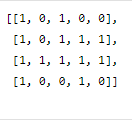New update is available. Click here to update.

# Maximum Area Square

Posted: 16 Dec, 2020
Difficulty: Moderate

## PROBLEM STATEMENT

#### For example, for the following grid:``````The area of the largest square submatrix with all 1s is 4.
``````
##### Input Format:
``````The first line contains an integer ‘T’ denoting the number of test cases. Then each test case follows.

The first input line of each test case contains two space-integers ‘N’ and ‘M’ representing the number of rows and columns of the grid, respectively.

From the second line of each test case, the next N lines represent the rows of the grid. Every row contains M single space-separated integers.
``````
##### Output Format:
``````For each test case, print the area of maximum size square sub-matrix with all 1s.

Print the output of each test case in a separate line.
``````
##### Note:
``````You are not required to print the expected output; it has already been taken care of. Just implement the function.
``````
##### Constraints:
``````1 <= T <= 100
1 <= N <= 50
1 <= M <= 50
0 <= MAT[i][j] <= 1

Time limit: 1 sec
``````
SIMILAR PROBLEMS

NINJA AND HAPPINESS

Posted: 9 Sep, 2022
Difficulty: Hard

DECODE STRING

Posted: 11 Sep, 2022
Difficulty: Moderate

Randomly Sorted

Posted: 13 Nov, 2022
Difficulty: Moderate

Search In A Sorted 2D Matrix

Posted: 23 Nov, 2022
Difficulty: Moderate

Spiral Matrix

Posted: 24 Nov, 2022
Difficulty: Easy